Function Repository Resource:

# PolyhedralGraphQ

Determine if a graph is polyhedral

Contributed by: Ed Pegg Jr
 ResourceFunction["PolyhedralGraphQ"][g] returns True if graph g is polyhedral.

## Details

A graph is polyhedral if it is both planar and 3-connected.
A graph is 3-connected if it will remain connected after the removal of any two edges.
If the edges in a graph can be interpreted as edges in a convex polyhedron, then the graph is polyhedral.

## Examples

### Basic Examples (3)

The tetrahedral graph is polyhedral:

 In:=Out=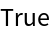The diamond graph is not polyhedral, since it can be disconnected by removing two edges:

 In:=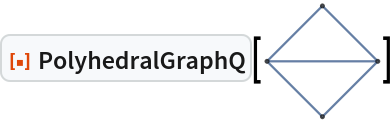Out=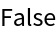The Petersen graph is not polyhedral since it is not planar:

 In:=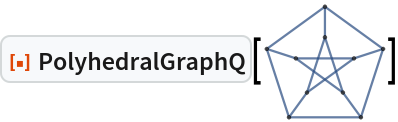Out=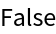### Neat Examples (2)

All polyhedral graphs with 1 to 9 vertices, grouped by number of vertices, in "g6" format:

 In:=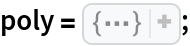Test the 257 polyhedral graphs with 8 vertices:

 In:=Out=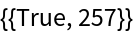## Version History

• 1.0.0 – 13 December 2022